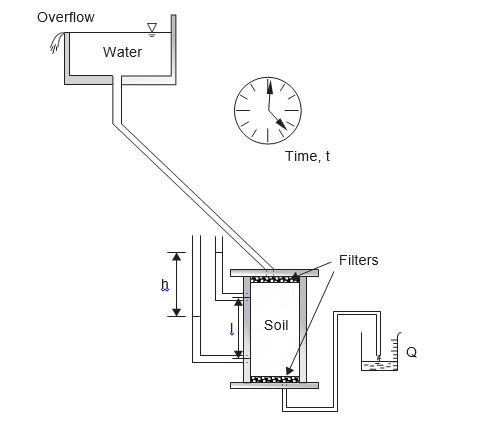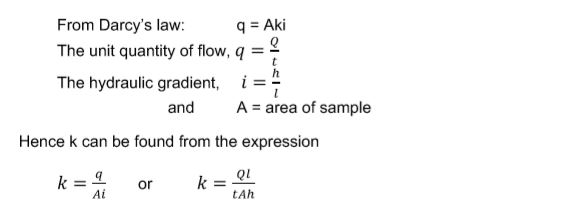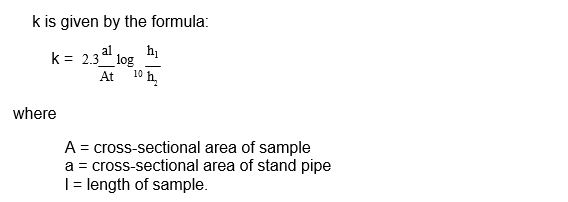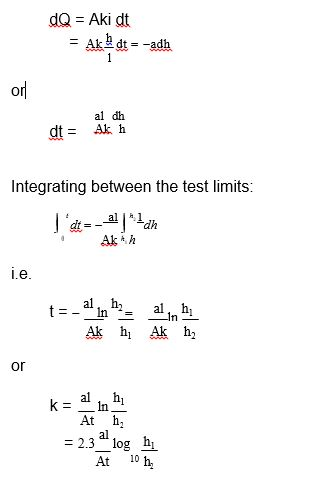Determination of permeability in the laboratory

How can web determinate the soil permeability? In this excerpt from the “Principle of Soil mechanics course”, Ian Smith gives some insight into this topic.

The test is described in BS 1377: Part 5 and the apparatus is shown in Figure 5.4. Water flows through the soil under a head which is kept constant by means of the overflow arrangement. The head loss, h, between two points along the length of the sample, distance l apart, is measured by means of a manometer.Figure 5.4. The constant head permeameter.A series of readings can be obtained from each test and an average value of k determined. The test is suitable for gravels and sands and could be used for many fill materials.

A sketch of the falling head permeameter is shown Figure 5.5. In this test, which is suitable for silts and some clays, the flow of water through the sample is measured at the inlet. The height, h1, in the stand-pipe is measured and the valve is then opened as a stop clock is started. After a measured time, t, the height to which the water level has fallen, h2, is determined.Figure 5.5. The falling head permeameter.During the test, the water in the stand-pipe falls from a height h1 to a final height h2.

Let h be the height at some time, t.

Consider a small time interval, dt, and let the change in the level of h during this time be –dh (negative as it is a drop in elevation).

The quantity of flow through the sample in time dt = –adh and is given the symbol dQ. Now:0 replies